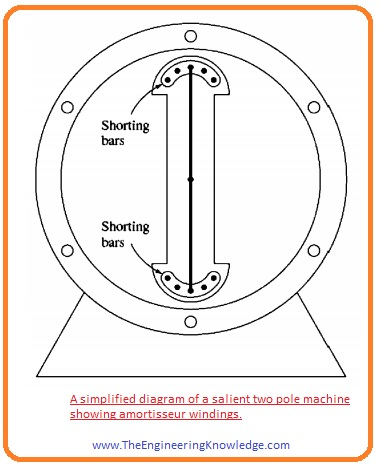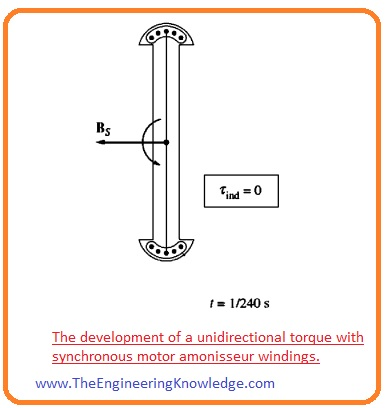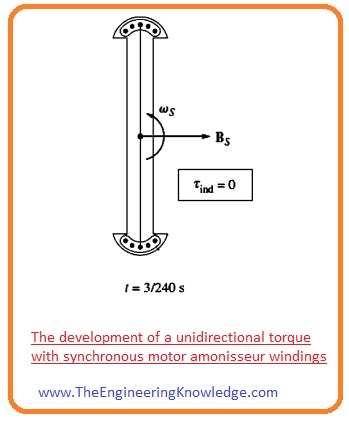Hello friends, I hope all of you are having fun in your life. In today’s tutorial, we are gonna have a look at Synchronous Motor Starting Methods. The running speed of the synchronous motor is equal to the speed of field rotating at stator that called synchronous speed. This speed depends on the frequency of input supply and no of poles of the motor. The formula for synchronous speed is Ns= (120f/P) where f is the frequency and P is the poles of the motor. From this equation, we can conclude that the speed of a motor can be varied by varying the frequency of input and poles of the motor.

There are numerous benefits of synchronous motor due to that it mostly used in industries like for power factor improvement. But it also has main drawback that it is not a self-starting motor like the induction motors. As in induction motor we just supply at the stator and it starts its operation while in the synchronous motor we need another extra supply at the rotor for excitation of the motor. The flux at the stator is revolving but at rotor due to DC source connection flux is constant. In today’s post, we will have a look at different starting techniques and their practical implementation. So let’s get started with Synchronous Motor Starting Method.

#### Synchronous Motor Starting Methods

• For a practical understanding of the issue that occurs in starting of motor we discuss a given below figure.• In this picture structure of sixty-hertz synchronous motor at starting conditions when input supply is provided to motor is shown.
``````
• The rotor of motor is static so the field at the rotor BR is also static. The stator’s field BS starts to revolve in the stator at synchronous speed.
• In the above figure, the circuit denoted as a tells about motor at (t=0) seconds when the rotor’s field BR and Bs are in a straight line. As we know torque induced equation.

tind = KBR x BS

• So the torque induced in the motor at t=0 according to torque equation will be zero.
• The circuit denoted as b explains the condition when the time (t = 1/240s). In this minor time interval motor hardly rotates, nonetheless, the direction of stator’s field is towards left side. According to torque equation, the direction of torque on the rotor is anticlockwise.
• The circuit in above figure denoted as c tells about the condition at (t=1/120s). at this time the rotor’s field and stator’s field are opposite to each other.
• The circuit denoted d explains the situation at (t=3/240), now both the field of rotor and stator are ninety degrees to each other and torque direction is clockwise.
• The last circuit that is denoted as e explains the situation at (t= 1/60s) at this time interval both the fields of stator and rotor are in a straight line.
• You can observe that from the above discussion that after completion of one electric rotation (cycle) first the torque direction was anticlockwise then clockwise and after completion of cycle average torque was 0.
• Due to these such conditions, motor starts vibration after every electrical rotation (cycle) and gets overheated.
• So there are 3 methods by which motor can be operated safely.
• Decrease the speed of rotation of field to a point at which rotor can easily interlock with it after half rotation of half-cycle of field. It is possible if we decrease the frequency of input supply connected motor.
• the second option is that rotate rotor with the exterior prime mover to the synchronous speed so it can interlock with the stator field after that remove the external prime mover and motor continues its operations.
• the thirds possibly for motor starting is that used damper windings it also called amortisseur windings, we will explain the effect of this winding with the detailed.
• Now we discuss these three-factors for the starting of synchronous motor with the detailed.

#### Synchronous Motor Starting by Reducing Electrical Frequency

• After that speed can again reach its normal operating frequency that is fifty or sixty hertz.
• Rectifier inverters and cycloconverter can be used for this process first to decreases frequency than increase it.
• With the invention of this circuitry nowadays it is very easy to vary the frequency from zero to desired value or overrated frequency.
• If we linked these frequency variable circuits with motor starting circuitry than it is very easy to change frequency at start of motor than increase according to system requirements.
• If motor is working at a speed less than the rated its internal generated voltage will also be less than normal value of voltage.
• With the decrement in internal generated voltage value, there will also decrease in terminal voltage of motor to maintain stator current at a safer level.
• In any variable frequency starting circuitry voltage will vary directly proportional to the frequency variations.

#### Synchronous Motor Starting with an External Prime Mover

• The second technique is to connect external starting motor with a synchronous motor to get its desired rated speed.
• When the motor gets its required speed then remove the exterior motor connected with the synchronous motor.
• When an external source is connected with motor removed then speed of rotation of shaft decreases, then the field of rotor BR lags the net field of a machine than generator behavior of motor ends and it starts to operate as a normal motor.
• After that load can be connected with the synchronous motor for normal operation.
• This entire process is not as absurd as it looks, as numerous synchronous motors are fragments of motor-generator sets, and the synchronous machine in the motor-generator set can start its operation with the other machine working as the starting motor.
• Similarly, the starting motor requires to decrease the inertia of the synchronous machine without a load, load connects with motor until it paralleled to the system.
• As the starting motor is used only to decrease inertia so its power rating should be less than the motor to start.

#### Synchronous Motor Starting by Using Amortisseur or Damper Windings

• The most famous technique for starting of synchronous motor is to use an Amortisseur winding, it also is known as damper
• A pole face with a set of damper winding is shown in given figure.• For understanding in a given figure, the salient pole rotor with having 2 poles consists of damper windings is shown.• This rotor displays a damper winding with the shorted bars at the end point of the 2 rotor pole faces coupled by wires. (it is not the practical method to construction of motor but it will help attractively to demonstrate the point of the damper windings).
• As stator field Bs rotates in an anticlockwise direction, due to its rotation voltage induced in damper (amortisseur) windings is given as.

eind = (v x B).l

• In this equation, the ‘V’ is the rotation speed of bars relative to field.
• ‘B’ is the flux density.
• ‘l’ is the length of conductive material in the field.
• So the bars at the top of rotor are rotating at 900 to field, so the resultant direction of the voltage generated is out of page. Correspondingly, the voltage generated is into the page at base (bottom) of rotor’s bar.
• Due to these two generated voltage-current produces at top bars out of page and bottom bars into the page, due to the windings field direction to the right side.
• According to the induced-torque equation, the resultant torque on the bars (and the rotor) is anticlockwise.

tind =kBw x Bs

• The given figure explains the conditions at (t =1/240s), in this situation the field of root has moved to ninety degrees but the rotor is almost at previous state, it is not possible for a rotor to move in this short time interval.• The voltage produces in this condition in the damper windings is out of page at base (bottom) of bars and into page at the top of bars.
• The resultant current starts to flow at the bottom bars out of page and into a page at the upper (top) bars, due to this field Bw is in the left side.
• The resultant torque for this situation is anti-clockwise and given as.

tind= kBw x Bs

• the given below figure explains the condition at (t=3/240s), at this time interval the induced torque is 0.• one thing you noticed that at some points torque is clockwise and at some points is anticlockwise and at some points is 0, but it always rotates in one direction.
• As the direction of net torque is unidirectional, so there is an increment in the speed of rotor.
• It is completely different from starting a synchronous motor with its usual IF, as in that case torque is first clockwise and then anticlockwise, so average torque is 0.
• But with damper windings torques follows one direction so it is not 0.
• Though the rotor speed of rotation increases, it can never approach synchronous speed.
• It is very simple to understand. Assume that a rotor is rotating at synchronous speed. So the speed of rotation of rotor will be equal to rotor rotation speed, so there is no comparative (relative) speed among the stator field Bs and rotor rotation.
• Due to absence of relative motion, there will be zero torque induces in windings. The resultant current will also 0 and field of windings will also 0.
• So there will be zero torque on rotor to rotate it.
• Though a rotor speed cannot equal to synchronous speed but it can be close to it.
• It can be close enough to synchronous speed that the steady IF can be turned on, and rotor will be aligned with the field of stator.
• In a practical motor, the field windings are not open when motor initiated. If these winnings’ are open then the high value of voltage will be generated in them during the initiated process of motor.
• If these (field windings) are short-circuited at the starting of motor no hazardous voltages are created, and the induced IF essentially delivers additional starting torque to the motor.

# Free SMT Assembly Monthly

New arrival for Aluminum boards, only \$2

## New users enjoy \$54 free coupons after signing up at JLCPCB successfully

\$54 coupons can also apply to 3D printing orders, for 3D printing special offer, it gets started at \$1